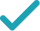Type a math problemSolve for xSolution Steps
Reduce the fraction to lowest terms by extracting and canceling out .
Convert decimal number to fraction . Reduce the fraction to lowest terms by extracting and canceling out .
Multiply times by multiplying numerator times numerator and denominator times denominator.
Do the multiplications in the fraction .
Reduce the fraction to lowest terms by extracting and canceling out .
Reduce the fraction to lowest terms by extracting and canceling out .
Convert decimal number to fraction . Reduce the fraction to lowest terms by extracting and canceling out .
Multiply times by multiplying numerator times numerator and denominator times denominator.
Do the multiplications in the fraction .
Least common multiple of and is . Convert and to fractions with denominator .
Since and have the same denominator, add them by adding their numerators.
Divide each term of by to get .
Use the distributive property to multiply by .
Convert decimal number to fraction .
Multiply times by multiplying numerator times numerator and denominator times denominator.
Do the multiplications in the fraction .
Least common multiple of and is . Convert and to fractions with denominator .
Since and have the same denominator, add them by adding their numerators.
Reduce the fraction to lowest terms by extracting and canceling out .
Subtract from both sides.
Convert decimal number to fraction . Reduce the fraction to lowest terms by extracting and canceling out .
Least common multiple of and is . Convert and to fractions with denominator .
Since and have the same denominator, subtract them by subtracting their numerators.
Subtract from to get .
Multiply both sides by , the reciprocal of . Since is , the inequality direction remains the same.
Express as a single fraction.
Multiply and to get .
Reduce the fraction to lowest terms by extracting and canceling out .
GraphGraph Inequality
Graph Both Sides in 2DGiving is as easy as 1, 2, 3
Get 1,000 points to donate to a school of your choice when you join Give With Bing
\frac{52.5+x}{48+50+48+52}\times 0.1+\frac{8}{10}\times 0.15+\frac{15}{30}\times 0.75>0.5
Add 28 and 24.5 to get 52.5.
\frac{52.5+x}{98+48+52}\times 0.1+\frac{8}{10}\times 0.15+\frac{15}{30}\times 0.75>0.5
Add 48 and 50 to get 98.
\frac{52.5+x}{146+52}\times 0.1+\frac{8}{10}\times 0.15+\frac{15}{30}\times 0.75>0.5
Add 98 and 48 to get 146.
\frac{52.5+x}{198}\times 0.1+\frac{8}{10}\times 0.15+\frac{15}{30}\times 0.75>0.5
Add 146 and 52 to get 198.
\frac{52.5+x}{198}\times 0.1+\frac{4}{5}\times 0.15+\frac{15}{30}\times 0.75>0.5
Reduce the fraction \frac{8}{10}\approx 0.8 to lowest terms by extracting and canceling out 2.
\frac{52.5+x}{198}\times 0.1+\frac{4}{5}\times \left(\frac{3}{20}\right)+\frac{15}{30}\times 0.75>0.5
Convert decimal number 0.15 to fraction \frac{15}{100}=0.15. Reduce the fraction \frac{15}{100}=0.15 to lowest terms by extracting and canceling out 5.
\frac{52.5+x}{198}\times 0.1+\frac{4\times 3}{5\times 20}+\frac{15}{30}\times 0.75>0.5
Multiply \frac{4}{5}=0.8 times \frac{3}{20}=0.15 by multiplying numerator times numerator and denominator times denominator.
\frac{52.5+x}{198}\times 0.1+\frac{12}{100}+\frac{15}{30}\times 0.75>0.5
Do the multiplications in the fraction \frac{4\times 3}{5\times 20}\approx 0.12.
\frac{52.5+x}{198}\times 0.1+\frac{3}{25}+\frac{15}{30}\times 0.75>0.5
Reduce the fraction \frac{12}{100}\approx 0.12 to lowest terms by extracting and canceling out 4.
\frac{52.5+x}{198}\times 0.1+\frac{3}{25}+\frac{1}{2}\times 0.75>0.5
Reduce the fraction \frac{15}{30}=0.5 to lowest terms by extracting and canceling out 15.
\frac{52.5+x}{198}\times 0.1+\frac{3}{25}+\frac{1}{2}\times \left(\frac{3}{4}\right)>0.5
Convert decimal number 0.75 to fraction \frac{75}{100}=0.75. Reduce the fraction \frac{75}{100}=0.75 to lowest terms by extracting and canceling out 25.
\frac{52.5+x}{198}\times 0.1+\frac{3}{25}+\frac{1\times 3}{2\times 4}>0.5
Multiply \frac{1}{2}=0.5 times \frac{3}{4}=0.75 by multiplying numerator times numerator and denominator times denominator.
\frac{52.5+x}{198}\times 0.1+\frac{3}{25}+\frac{3}{8}>0.5
Do the multiplications in the fraction \frac{1\times 3}{2\times 4}=0.375.
\frac{52.5+x}{198}\times 0.1+\frac{24}{200}+\frac{75}{200}>0.5
Least common multiple of 25 and 8 is 200. Convert \frac{3}{25}=0.12 and \frac{3}{8}=0.375 to fractions with denominator 200.
\frac{52.5+x}{198}\times 0.1+\frac{24+75}{200}>0.5
Since \frac{24}{200}=0.12 and \frac{75}{200}=0.375 have the same denominator, add them by adding their numerators.
\frac{52.5+x}{198}\times 0.1+\frac{99}{200}>0.5
Add 24 and 75 to get 99.
\left(\frac{35}{132}+\frac{1}{198}x\right)\times 0.1+\frac{99}{200}>0.5
Divide each term of 52.5+x by 198 to get \frac{35}{132}+\frac{1}{198}x.
\frac{7}{264}+\frac{1}{198}x\times 0.1+\frac{99}{200}>0.5
Use the distributive property to multiply \frac{35}{132}+\frac{1}{198}x by 0.1.
\frac{7}{264}+\frac{1}{198}x\times \left(\frac{1}{10}\right)+\frac{99}{200}>0.5
Convert decimal number 0.1 to fraction \frac{1}{10}=0.1.
\frac{7}{264}+\frac{1\times 1}{198\times 10}x+\frac{99}{200}>0.5
Multiply \frac{1}{198}\approx 0.005050505 times \frac{1}{10}=0.1 by multiplying numerator times numerator and denominator times denominator.
\frac{7}{264}+\frac{1}{1980}x+\frac{99}{200}>0.5
Do the multiplications in the fraction \frac{1\times 1}{198\times 10}\approx 0.000505051.
\frac{175}{6600}+\frac{1}{1980}x+\frac{3267}{6600}>0.5
Least common multiple of 264 and 200 is 6600. Convert \frac{7}{264}\approx 0.026515152 and \frac{99}{200}=0.495 to fractions with denominator 6600.
\frac{175+3267}{6600}+\frac{1}{1980}x>0.5
Since \frac{175}{6600}\approx 0.026515152 and \frac{3267}{6600}=0.495 have the same denominator, add them by adding their numerators.
\frac{3442}{6600}+\frac{1}{1980}x>0.5
Add 175 and 3267 to get 3442.
\frac{1721}{3300}+\frac{1}{1980}x>0.5
Reduce the fraction \frac{3442}{6600}\approx 0.521515152 to lowest terms by extracting and canceling out 2.
\frac{1}{1980}x>0.5-\frac{1721}{3300}
Subtract \frac{1721}{3300}\approx 0.521515152 from both sides.
\frac{1}{1980}x>\frac{1}{2}-\frac{1721}{3300}
Convert decimal number 0.5 to fraction \frac{5}{10}=0.5. Reduce the fraction \frac{5}{10}=0.5 to lowest terms by extracting and canceling out 5.
\frac{1}{1980}x>\frac{1650}{3300}-\frac{1721}{3300}
Least common multiple of 2 and 3300 is 3300. Convert \frac{1}{2}=0.5 and \frac{1721}{3300}\approx 0.521515152 to fractions with denominator 3300.
\frac{1}{1980}x>\frac{1650-1721}{3300}
Since \frac{1650}{3300}=0.5 and \frac{1721}{3300}\approx 0.521515152 have the same denominator, subtract them by subtracting their numerators.
\frac{1}{1980}x>-\frac{71}{3300}
Subtract 1721 from 1650 to get -71.
x>-\frac{71}{3300}\times 1980
Multiply both sides by 1980, the reciprocal of \frac{1}{1980}\approx 0.000505051. Since \frac{1}{1980}\approx 0.000505051 is >0, the inequality direction remains the same.
x>\frac{-71\times 1980}{3300}
Express -\frac{71}{3300}\times 1980\approx -42.6 as a single fraction.
x>\frac{-140580}{3300}
Multiply -71 and 1980 to get -140580.
x>-\frac{213}{5}
Reduce the fraction \frac{-140580}{3300}\approx -42.6 to lowest terms by extracting and canceling out 660.